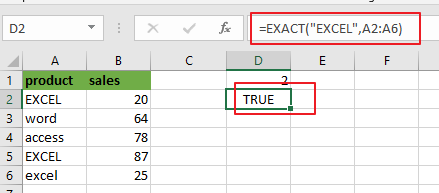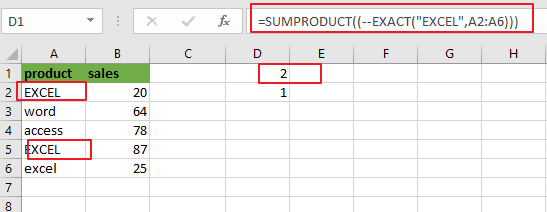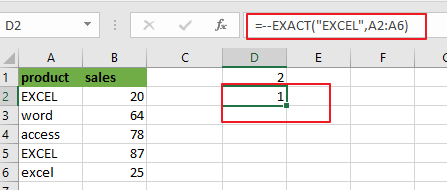# How to Count Cells equals to Cells with Case Sensitive in Excel

This post will guide you how to count the number of cells equal to a specific value in a given range cells with case sensitive using a formula in Excel 2013/2016.you can easily to count cells equal to a specific value without case sensitive through COUNTIF function. But if there is an easy way to count cells equal to a cell values in a selected range of cells with case sensitive in Excel.

## Count Cells equals to Cells with Case Sensitive

Assuming that you want to count cells that contain value “`EXCEL`” defined in a selected range(A1:B6), taking into account upper and lower case. In this case, you can use the SUMPRODUCT function combining with the EXACT function to count cells exactly equal to with case sensitive in your worksheet.

Enter the following formula in a blank cell, and press Enter key:

=SUMPRODUCT((–EXACT(“EXCEL”,A2:A6)))Note: the above formula provides a case-sensitive count of a text string value “EXCEL”. A2:A6 is the data range that you want to use. And “EXCEL” is the specific value that you want to count in range A2:A6. You need to change them as you need.

Now Let’s see how this formula works:

The EXACT function has two arguments. When those two arguments match exactly, it returns TRUE value. Otherwise, it returns FALSE value.

=EXACT(“EXCEL”,A2:A6)This formula will compare one text value “EXCEL” with each cell values in range A2:A6. Actually, It will return an array result similar to the following values:

{TRUE,FALSE,FALSE,TRUE,FALSE}

Then you need to use a double-negative(–) to convert TRUE and FALSE values into “1” or “0”. And the result is changed as the below array:

{1,0,0,1,0}The above array result will be passed into the SUMPRODUCT function as its argument, and sums all values in the array result. It should return number 2.

### Related Functions

• Excel SUMPRODUCT function
The Excel SUMPRODUCT function multiplies corresponding components in the given one or more arrays or ranges, and returns the sum of those products. The syntax of the SUMPRODUCT function is as below:= SUMPRODUCT (array1,[array2],…)…
• Excel EXACT function
The Excel EXACT function compares if two text strings are the same and returns TRUE if they are the same, Or, it will return FALSE.The syntax of the EXACT function is as below:= EXACT (text1,text2)…